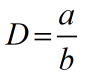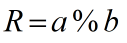Request a Tool

Long Division Calculator

Long Division Calculator is use to calculate division in decimal and remainder form.

Long Division
0

Formula• D = Division
• a = Values of Divident
• b = Values of Divisor

Defination / Uses

Long division is a division procedure in mathematics that is ideal for dividing multi-digit Arabic numerals and is easy to accomplish by hand. It simplifies a division problem by breaking it down into smaller chunks.

Long Division
0

Formula• R = Remainder
• a = Values of Dividend
• b = Values of Divisor

Defination / Uses

The basic remainder formula is Dividend/Divisor = Quotient + Remainder/Divisor in the abstract. We may get another useful variant of the remaining formula by multiplying through by the Divisor: Quotient*Divisor + Remainder = Dividend.

Welcome to the long division calculator, a tool that will assist you in learning how to divide decimals via long division. Find the answer as a quotient with a remainder by dividing two numbers, a dividend and a divisor. Learn how to solve long division problems with remainders, or practise your own long division problems and check your solutions using this calculator. One of two methods for doing long division by hand is long division with remainders. Finding a quotient answer using a decimal is a little easier than solving a division problem. Use our Long Division with Decimals Calculator if you need to do long division with decimals.